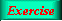Lecture 4 - Page 9 : 34
 Functional Programming in SchemeHigher-order Functions * Introduction to higher-order functions Higher-order functions Some simple and general higher-order functions Linear search in lists Generation of list selectors * Mapping and filtering Classical higher-order functions: Overview Mapping The mapping function Examples of mapping Filtering The filtering function Examples of filtering * Reduction and zipping Reduction The reduction functions Accumulation Zipping The zipping function * Currying The idea of currying Currying in Scheme Examples of currying Ad hoc currying in Scheme (1) Ad hoc currying in Scheme (2) * Web related higher-order functions HTML mirror generation HTML mirror usage examples Making tables with the real mirror Tables with higher-order functions HTML element modifications The function simple-html-table The XHTML mirror in LAML Generation of a leq predicate from enumeration
 The mapping function It is now time to study the implementation of the mapping function. We program a function called mymap in order not to redefine Scheme's own mapping function (a standard function in all Scheme implementations).
 A possible implementation of map, called mymap:
 ```(define (mymap f lst) (if (null? lst) '() (cons (f (car lst)) (mymap f (cdr lst)))))```

An implementation of map. This is not a good implementation because the recursive call is not a tail call. We leave it as an exercise to make a memory efficient implementation with tail recursion - see the exercise below.Iterative mapping functionTable exercise: transposing, row elimination, and column elimination.